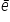# Covalent Bond

Covalent Bond:-Lewis – Longmuir Concept:-

Langmuir   in. 1919 improved Lewis Concept.

Covalent Bond=> is the bond made between two atoms by mutual sharing of electrons between them so an to complete their octets (or duplet in case of x, Be and Li)

Covalent bond can be single. Double or Triple, Double and triple bonds are known as multiple bonds.

• When 1pair is shared between 2 atoms then Single bond is formed.
• When 2pair is shared between 2 atoms then Double bond is formed.
• When 3pair is shared between 2 atoms then Triple bond is formed.

Shown by (-), (=) or ( )

Example=>

Conditions for covalent bond formation=>Covalent bond is made between similar atoms or atoms have if small diff. of electron negativities

=>Covalence=> It is equal to the n: ofcontributed by an atom for share with other atom.

Characteristics of covalent compound=>

• These except as solids, liquids and gases.
• Lower.  Biolong  Point (except Diamonds, etc.)
• Insoluble in Water.
• They are directional in nature.

Drawbacks of concept of covalent bond

(i)   It would not explain the force of attraction between two atoms (ii) would not explain shapes and release of En. during their formation.

Formal Charge=> or (F.C)

F.C on an atom is Molecule or ion = [total number of valence Ä“ in free atoms] – [total number of non-bonding Ä“] – ½

F. C = V – L – ½ S [number of Ä“ shared i.e. bonding Ä“ ]

V => valence Ä“

L => Lone pairs   S=> Shared Ä“

Q.      Calculate F.C. On each atom in O3

Formal charge on O1 = 6 – 4 – ½  x  4 = Zero

Formal charge on O2 = 6 – 2 – ½ x 6 = +1

Formal charge on O3 = 6 – 6 – ½ x 2 = -1

Related Keywords